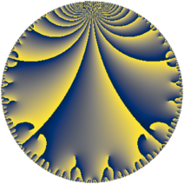# Properties

 Label 18.7.dLevel $18$ Weight $7$ Character orbit 18.d Rep. character $\chi_{18}(5,\cdot)$ Character field $\Q(\zeta_{6})$ Dimension $12$ Newform subspaces $1$ Sturm bound $21$ Trace bound $0$

# Related objects

## Defining parameters

 Level: $$N$$ $$=$$ $$18 = 2 \cdot 3^{2}$$ Weight: $$k$$ $$=$$ $$7$$ Character orbit: $$[\chi]$$ $$=$$ 18.d (of order $$6$$ and degree $$2$$) Character conductor: $$\operatorname{cond}(\chi)$$ $$=$$ $$9$$ Character field: $$\Q(\zeta_{6})$$ Newform subspaces: $$1$$ Sturm bound: $$21$$ Trace bound: $$0$$

## Dimensions

The following table gives the dimensions of various subspaces of $$M_{7}(18, [\chi])$$.

Total New Old
Modular forms 40 12 28
Cusp forms 32 12 20
Eisenstein series 8 0 8

## Trace form

 $$12 q - 42 q^{3} + 192 q^{4} + 432 q^{5} - 144 q^{6} + 240 q^{7} + 2190 q^{9} + O(q^{10})$$ $$12 q - 42 q^{3} + 192 q^{4} + 432 q^{5} - 144 q^{6} + 240 q^{7} + 2190 q^{9} + 378 q^{11} + 384 q^{12} + 1680 q^{13} - 4752 q^{14} - 10872 q^{15} - 6144 q^{16} - 2976 q^{18} - 2820 q^{19} + 13824 q^{20} + 24876 q^{21} - 3600 q^{22} - 76248 q^{23} - 6144 q^{24} + 8094 q^{25} + 127008 q^{27} + 15360 q^{28} + 97092 q^{29} + 34272 q^{30} + 21480 q^{31} - 246258 q^{33} - 27360 q^{34} + 38208 q^{36} - 25536 q^{37} + 97632 q^{38} + 42204 q^{39} - 410562 q^{41} - 222144 q^{42} + 71430 q^{43} + 13716 q^{45} - 135072 q^{46} + 347652 q^{47} + 55296 q^{48} - 135954 q^{49} + 311040 q^{50} + 336402 q^{51} - 53760 q^{52} - 173520 q^{54} + 580392 q^{55} - 152064 q^{56} - 522282 q^{57} + 159264 q^{58} + 369738 q^{59} - 170496 q^{60} + 135744 q^{61} - 103800 q^{63} - 393216 q^{64} - 753840 q^{65} + 909216 q^{66} - 289938 q^{67} + 744768 q^{68} + 2059272 q^{69} + 155952 q^{70} - 374784 q^{72} - 977700 q^{73} - 2197152 q^{74} - 2115342 q^{75} - 45120 q^{76} - 159192 q^{77} - 631488 q^{78} - 764796 q^{79} - 1428282 q^{81} + 1073088 q^{82} + 396900 q^{83} + 1441536 q^{84} + 1619568 q^{85} + 3264624 q^{86} + 3072636 q^{87} + 115200 q^{88} - 1987200 q^{90} + 355584 q^{91} - 2439936 q^{92} - 2526576 q^{93} - 736848 q^{94} - 2089260 q^{95} - 49152 q^{96} - 38874 q^{97} + 4398804 q^{99} + O(q^{100})$$

## Decomposition of $$S_{7}^{\mathrm{new}}(18, [\chi])$$ into newform subspaces

Label Dim $A$ Field CM Traces $q$-expansion
$a_{2}$ $a_{3}$ $a_{5}$ $a_{7}$
18.7.d.a $12$ $4.141$ $$\mathbb{Q}[x]/(x^{12} + \cdots)$$ None $$0$$ $$-42$$ $$432$$ $$240$$ $$q-\beta _{3}q^{2}+(-6+4\beta _{1}+\beta _{2}-\beta _{3}+\beta _{7}+\cdots)q^{3}+\cdots$$

## Decomposition of $$S_{7}^{\mathrm{old}}(18, [\chi])$$ into lower level spaces

$$S_{7}^{\mathrm{old}}(18, [\chi]) \cong$$ $$S_{7}^{\mathrm{new}}(9, [\chi])$$$$^{\oplus 2}$$